# Find average value over number of bars?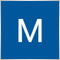21

I'm trying to write a custom indicator that relies on finding the average value over a past number of bars, but I'm having trouble figuring out how to write the function, although it should be simple, but I don't see an "average" function prebuilt into mql4 and I don't think I would need to save each value into it's own variable and divide by the number of variables manually. There has to be a better way than that. For instance, if I want to find the average value of the Close price minus the Open prices over the past x number of bars, how would I be able to do that? Any ideas on writing a simple script that doesn't make me save each bar value into it's own variable, which would be cumbersome if I had more than say 10 bars, and then manually divide by the number of bars?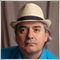11340

MPW Capital: I'm trying to write a custom indicator that relies on finding the average value over a past number of bars, but I'm having trouble figuring out how to write the function, although it should be simple, but I don't see an "average" function prebuilt into mql4 and I don't think I would need to save each value into it's own variable and divide by the number of variables manually. There has to be a better way than that. For instance, if I want to find the average value of the Close price minus the Open prices over the past x number of bars, how would I be able to do that? Any ideas on writing a simple script that doesn't make me save each bar value into it's own variable, which would be cumbersome if I had more than say 10 bars, and then manually divide by the number of bars?

It's called a Simple Moving Average and is the most basic of indicators build into every platform I know (and that includes MetaTrader).

The MQL4 function for it (and several other types of moving averages) is "iMA()". If you need to apply it to custom data, then use "iMAOnArray()".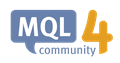iMA - Technical Indicators - MQL4 Reference
• docs.mql4.com
iMA - Technical Indicators - MQL4 Reference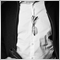2673

Here is what you need to calculate the mean of a series.

A variable to sum up the values.
An array with the length of the amount of values you want to consider.

Here is what you need to do:
You use the array as a ring buffer.

On a new value/period:
You push the pointer of your ring buffer by one.

You take the value stored at the ring buffers new position and subtract it from your sum variable.

You add the new value to your sum variable and store it in the ring buffer.

Note: you need to do this every time you >update< the value in case of a change. (Subtract, add, store)

To get your result, you divide your sum by the length of the ring buffer (elements in the buffer).

That will give you a concept where you can create any mean value from any input you want to feed into it. It's very efficient and has a deterministic calculation speed, no matter the length of your mean.

Hope this was understandable.415

MPW Capital:

...I would need to save each value into it's own variable and divide by the number of variables manually. There has to be a better way than that....

I cannot comment on MQL4, but if you look in the MQL5 official library, you will see that's basically how they do it using an array and a loop.

So no harm in copying that way if there is no function in MQL457

MPW Capital:

I'm trying to write a custom indicator that relies on finding the average value over a past number of bars, but I'm having trouble figuring out how to write the function, although it should be simple, but I don't see an "average" function prebuilt into mql4 and I don't think I would need to save each value into it's own variable and divide by the number of variables manually. There has to be a better way than that. For instance, if I want to find the average value of the Close price minus the Open prices over the past x number of bars, how would I be able to do that? Any ideas on writing a simple script that doesn't make me save each bar value into it's own variable, which would be cumbersome if I had more than say 10 bars, and then manually divide by the number of bars?

ArraySetAsSeries(Buf_Par,true);

double AveP = iMAOnArray(Buf_Par,0, 15, 0,MODE_SMA, i);

Buf_AveP[i] = AveP;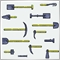1275

You need to find out how for loops and arrays work or you leave the work to somebody else.

It is not hard if you know how but it is real work to find that out.